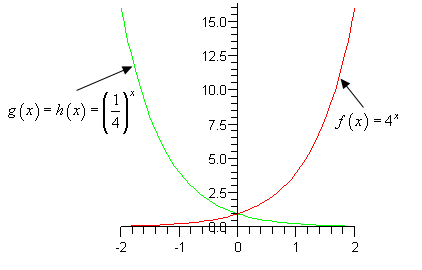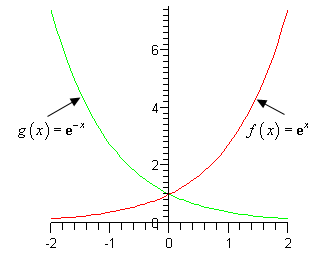Paul's Online Notes
Home / Algebra Trig Review / Exponentials & Logarithms / Basic Exponential Functions
Show Mobile Notice Show All Notes Hide All Notes
Mobile Notice
You appear to be on a device with a "narrow" screen width (i.e. you are probably on a mobile phone). Due to the nature of the mathematics on this site it is best views in landscape mode. If your device is not in landscape mode many of the equations will run off the side of your device (should be able to scroll to see them) and some of the menu items will be cut off due to the narrow screen width.

### Section 3.1 : Basic Exponential Functions

First, let’s recall that for $$b > 0$$ and $$b \ne 1$$ an exponential function is any function that is in the form

$f\left( x \right) = {b^x}$

We require $$b \ne 1$$ to avoid the following situation,

$f\left( x \right) = {1^x} = 1$

So, if we allowed $$b = 1$$ we would just get the constant function, 1.

We require $$b > 0$$ to avoid the following situation,

$f\left( x \right) = {\left( { - 4} \right)^x}\hspace{0.25in}\hspace{0.25in} \Rightarrow \,\hspace{0.25in}\,\,\,\,f\left( {\frac{1}{2}} \right) = {\left( { - 4} \right)^{\frac{1}{2}}} = \sqrt { - 4}$

By requiring $$b > 0$$ we don’t have to worry about the possibility of square roots of negative numbers.

Show All Solutions Hide All Solutions

1. Evaluate $$f\left( x \right) = {4^x}$$, $$g\left( x \right) = {\left( \frac{1}{4}} \right)^x$$ and $$h\left( x \right) = {4^{ - x}}$$ at $$x = - 2, - 1,0,1,2$$.
Show Solution

The point here is mostly to make sure you can evaluate these kinds of functions. So, here’s a quick table with the answers.

$$\displaystyle x = - 2$$ $$\displaystyle x = - 1$$ $$\displaystyle x = 0$$ $$\displaystyle x = 1$$ $$\displaystyle x = 2$$
$$\displaystyle f\left( x \right)$$ $$\displaystyle f\left( { - 2} \right) = \frac{1}{{16}}$$ $$\displaystyle f\left( { - 1} \right) = \frac{1}{4}$$ $$\displaystyle f\left( 0 \right) = 1$$ $$\displaystyle f\left( 1 \right) = 4$$ $$\displaystyle f\left( 2 \right) = 16$$
$$\displaystyle g\left( x \right)$$ $$\displaystyle g\left( { - 2} \right) = 16$$ $$\displaystyle g\left( { - 1} \right) = 4$$ $$\displaystyle g\left( 0 \right) = 1$$ $$\displaystyle g\left( 1 \right) = \frac{1}{4}$$ $$\displaystyle g\left( 2 \right) = \frac{1}{{16}}$$
$$\displaystyle h\left( x \right)$$ $$\displaystyle h\left( { - 2} \right) = 16$$ $$\displaystyle h\left( { - 1} \right) = 4$$ $$\displaystyle h\left( 0 \right) = 1$$ $$\displaystyle h\left( 1 \right) = \frac{1}{4}$$ $$\displaystyle h\left( 2 \right) = \frac{1}{{16}}$$

Notice that the last two rows give exactly the same answer. If you think about it that should make sense because,

$g\left( x \right) = {\left( {\frac{1}{4}} \right)^x} = \frac{{{1^x}}}{{{4^x}}} = \frac{1}{{{4^x}}} = {4^{ - x}} = h\left( x \right)$
2. Sketch the graph of $$f\left( x \right) = {4^x}$$, $$g\left( x \right) = {\left( \frac{1}{4}} \right)^x$$ and $$h\left( x \right) = {4^{ - x}}$$ on the same axis system.
Show Solution

Note that we only really need to graph $$f\left( x \right)$$ and $$g\left( x \right)$$ since we showed in the previous Problem that $$g\left( x \right) = h\left( x \right)$$. Note as well that there really isn’t too much to do here. We found a set of values in Problem 1 so all we need to do is plot the points and then sketch the graph. Here is the sketch,3. List as some basic properties for $$f\left( x \right) = {b^x}$$.
Show Solution

Most of these properties can be seen in the sketch in the previous Problem.

(a) $$f\left( x \right) = {b^x} > 0$$ for every $$x$$. This is a direct consequence of the requirement that $$b > 0$$.

(b) For any $$b$$ we have $$f\left( 0 \right) = {b^0} = 1$$.

(c) If $$b > 1$$ ($$f\left( x \right) = {4^x}$$ above, for example) we see that $$f\left( x \right) = {b^x}$$ is an increasing function and that,

$f\left( x \right) \to \infty {\mbox{ as }}x \to \infty \hspace{0.5in}{\rm{and}}\hspace{0.5in}f\left( x \right) \to 0{\mbox{ as }}x \to - \infty$

(d) If $$0 < b < 1$$ ($$g\left( x \right) = {\left( {\frac{1}{4}} \right)^x}$$ above, for example) we see that $$f\left( x \right) = {b^x}$$ is an decreasing function and that,

$f\left( x \right) \to 0{\mbox{ as }}x \to \infty \hspace{0.5in}{\rm{and}}\hspace{0.5in}f\left( x \right) \to \infty {\mbox{ as }}x \to - \infty$

Note that the last two properties are very important properties in many Calculus topics and so you should always remember them!

4. Evaluate $$f\left( x \right) = {{\bf{e}}^x}$$, $$g\left( x \right) = {{\bf{e}}^{ - x}}$$ and $$h\left( x \right) = 5{{\bf{e}}^{1 - 3x}}$$ at $$x = - 2, - 1,0,1,2$$.
Show Solution

Again, the point of this problem is to make sure you can evaluate these kinds of functions. Recall that in these problems $$e$$ is not a variable it is a number! In fact, $${\bf{e}} = 2.718281828 \ldots$$

When computing $$h\left( x \right)$$ make sure that you do the exponentiation BEFORE multiplying by 5.

$$\displaystyle x = - 2$$ $$\displaystyle x = - 1$$ $$\displaystyle x = 0$$ $$\displaystyle x = 1$$ $$\displaystyle x = 2$$
$$\displaystyle f\left( x \right)$$ $$\displaystyle 0.135335$$ $$\displaystyle 0.367879$$ $$\displaystyle 1$$ $$\displaystyle 2.718282$$ $$\displaystyle 7.389056$$
$$\displaystyle g\left( x \right)$$ $$\displaystyle 7.389056$$ $$\displaystyle 2.718282$$ $$\displaystyle 1$$ $$\displaystyle 0.367879$$ $$\displaystyle 0.135335$$
$$\displaystyle h\left( x \right)$$ $$\displaystyle 5483.166$$ $$\displaystyle 272.9908$$ $$\displaystyle 13.59141$$ $$\displaystyle 0.676676$$ $$\displaystyle 0.033690$$

5. Sketch the graph of $$f\left( x \right) = {{\bf{e}}^x}$$ and $$g\left( x \right) = {{\bf{e}}^{ - x}}$$.
Show Solution

As with the other “sketching” problem there isn’t much to do here other than use the numbers we found in the previous example to make the sketch. Here it is,Note that from these graphs we can see the following important properties about $$f\left( x \right) = {{\bf{e}}^x}$$ and $$g\left( x \right) = {{\bf{e}}^{ - x}}$$.

\begin{align*} & {{\bf{e}}^x} \to \infty {\mbox{ as }}x \to \infty & \hspace{0.5in} & {\rm{and}} & \hspace{0.5in} & {{\bf{e}}^x} \to 0{\mbox{ as }}x \to - \infty \\ & {{\bf{e}}^{ - x}} \to 0{\mbox{ as }}x \to \infty & \hspace{0.25in} & {\rm{and}} & \hspace{0.25in} & {{\bf{e}}^{ - x}} \to \infty {\mbox{ as }}x \to - \infty \end{align*}

These properties show up with some regularity in a Calculus course and so should be remembered.